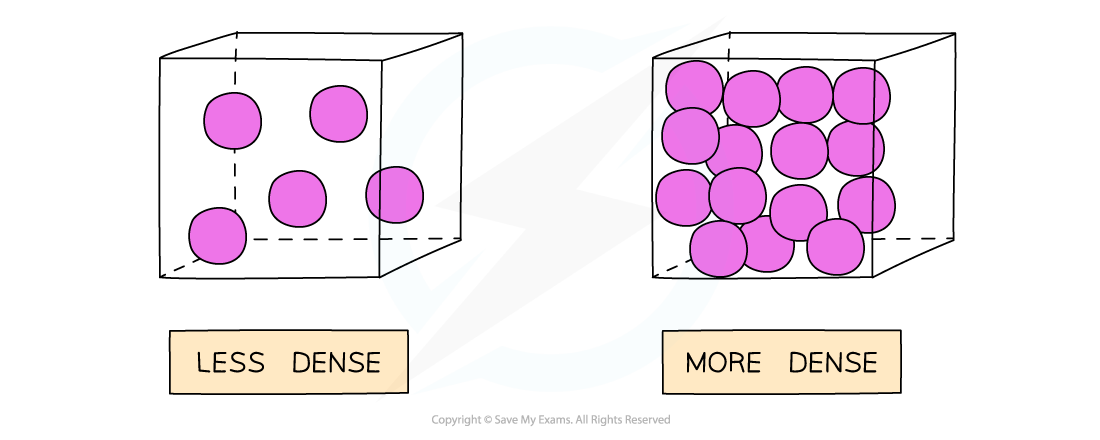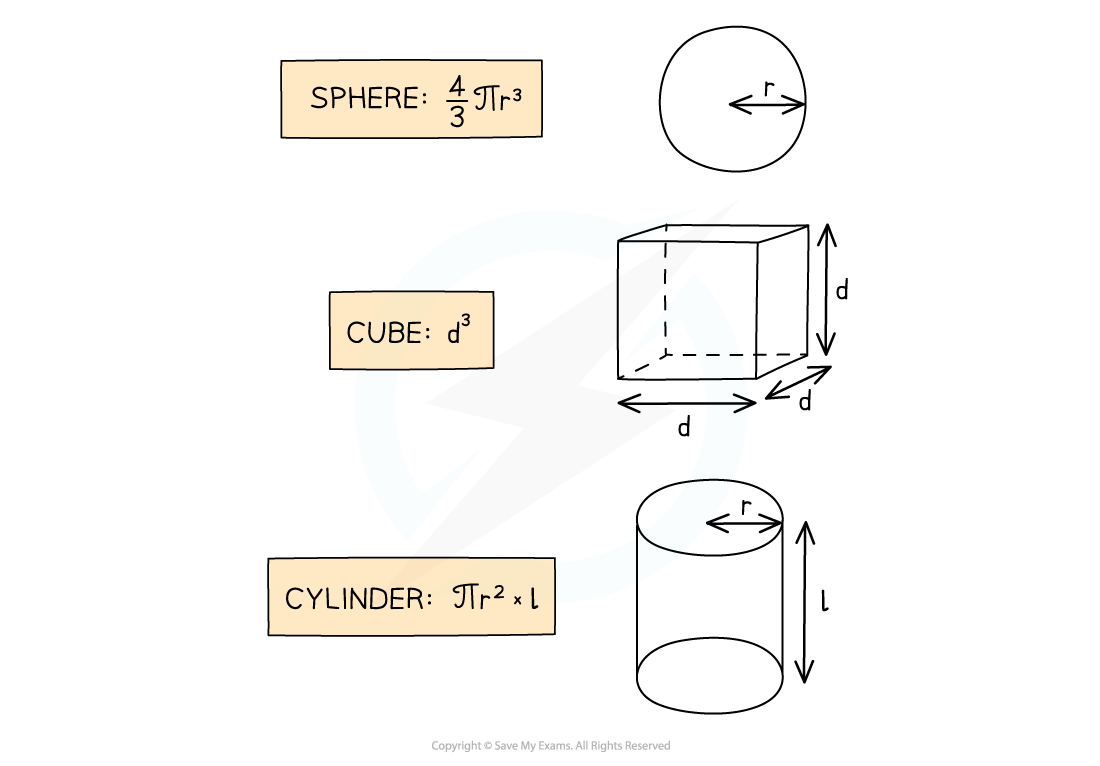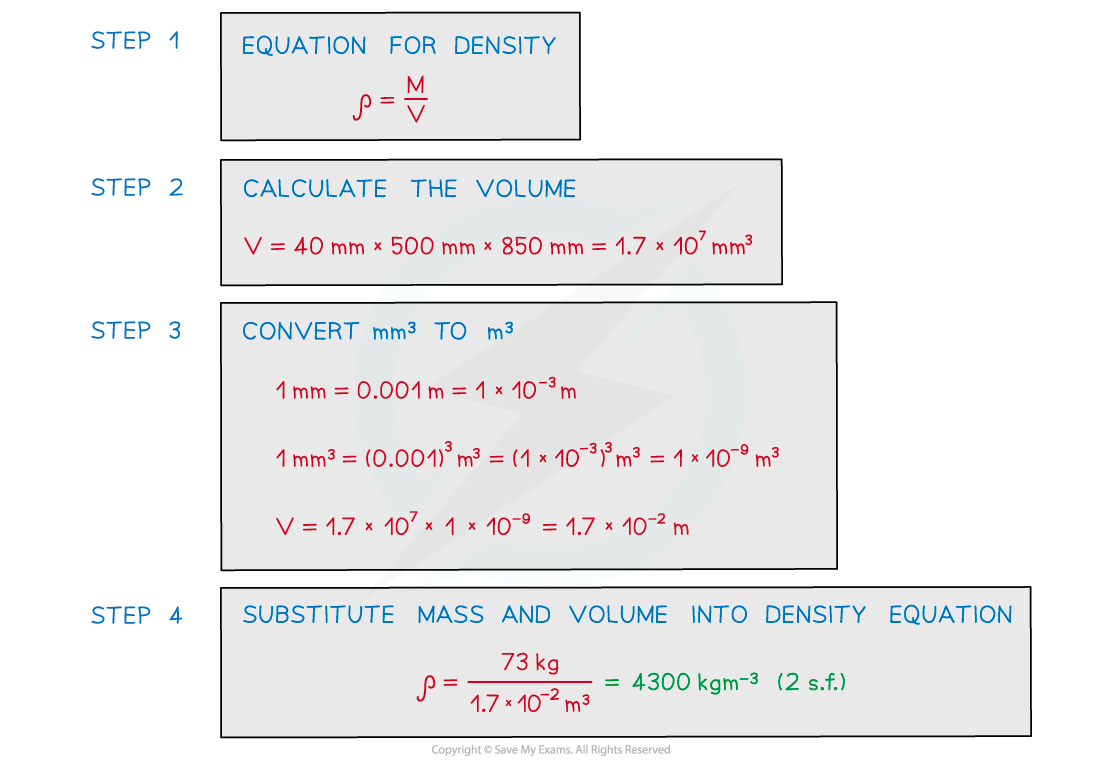# AQA A Level Physics复习笔记4.7.1 Density

### Density

• Density is the mass per unit volume of an object
• Objects made from low-density materials typically have a lower mass
• For example, a balloon is less dense than a small bar of lead despite occupying a larger volume

• The units of density depend on the units used for mass and volume:
• If the mass is measured in g and volume in cm3, then the density will be in g / cm3
• If the mass is measured in kg and volume in m3, then the density will be in kg / m3Gases are less dense than a solid

• The volume of an object may not always be given directly, but can be calculated with the appropriate equation depending on the object’s shapeVolumes of common 3D shapes

#### Worked Example

A paving slab has a mass of 73 kg and dimensions 40 mm × 500 mm × 850 mm.

Calculate the density, in kg m-3 of the material from which the paving slab is made.#### Exam Tip

• When converting a larger unit to a smaller one, you multiply (×)
• E.g. 125 m = 125 × 100 = 12 500 cm

• When you convert a smaller unit to a larger one, you divide (÷)
• E.g. 5 g = 5 / 1000 = 0.005 or 5 × 10-3 kg

• When dealing with squared or cubic conversions, cube or square the conversion factor too
• E.g. 1 mm3 = 1 / (1000)3 = 1 × 10-9 m3
• E.g. 1 cm3 = 1 / (100)3 = 1 × 10-6 m3